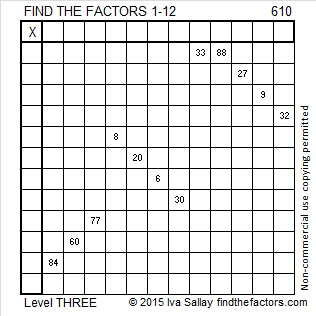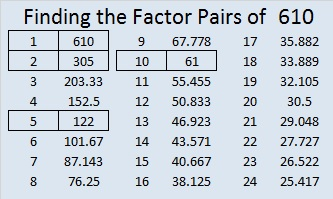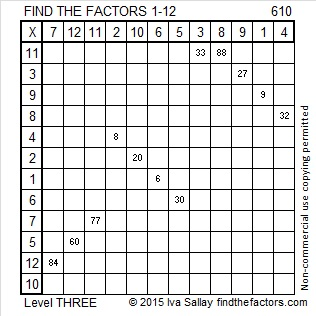# 610 and Level 3

The first 17 Fibonacci numbers are 1, 1, 2, 3, 5, 8, 13, 21, 34, 55, 89, 144, 233, 377, 610, 987, 1597. Notice that 610 is the 15th Fibonacci number.

There is a fascinating relationship between some of the Fibonacci numbers and some of the Markov numbers. 610 is the 12th Markov number. Get out your calculator and satisfy yourself that the following two Diophantine equations involving Fibonacci/Markov numbers are true:

1² + 233² + 610² = 3(1)(233)(610)

1² + 610² + 1597² = 3(1)(610)(1597)

Here is a fascinating fact I learned from twitter:

Starting with 5, every other Fibonacci number would be 5, 13, 34, 89, 233, 610, 1597, . . .

610 is on that list. What could be the integer sides of a right triangle with 610 as the hypotenuse?

There are actually FOUR such triangles, namely. . .

• 110-600-610
• 272-546-610
• 414-448-610
• 366-488-610

None of those are primitives, but it is a great list nonetheless!Print the puzzles or type the solution on this excel file: 12 Factors 2015-09-07

—————————————————————————————————

• 610 is a composite number.
• Prime factorization: 610 = 2 x 5 x 61
• The exponents in the prime factorization are 1, 1, and 1. Adding one to each and multiplying we get (1 + 1)(1 + 1)(1 + 1) = 2 x 2 x 2 = 8. Therefore 610 has exactly 8 factors.
• Factors of 610: 1, 2, 5, 10, 61, 122, 305, 610
• Factor pairs: 610 = 1 x 610, 2 x 305, 5 x 122, or 10 x 61
• 610 has no square factors that allow its square root to be simplified. √610 ≈ 24.698178.—————————————————————————————————

A Logical Approach to solve a FIND THE FACTORS puzzle: Find the column or row with two clues and find their common factor. Write the corresponding factors in the factor column (1st column) and factor row (top row).  Because this is a level three puzzle, you have now written a factor at the top of the factor column. Continue to work from the top of the factor column to the bottom, finding factors and filling in the factor column and the factor row one cell at a time as you go.